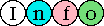## The Chances Of Winning The UK National Lottery

• The Jackpot - 6 Numbers (Typical prize pool: Sat - £5m, Wed - £3m)
6 numbers are drawn at random from the set of integers between 1 and 49, which means there are 49!/(6!*(49-6)!) combinations of numbers (the draw order doesn't matter). The means that the jackpot chance is 1 in 13,983,816 or approximately 1 in 14 million.

• 5 Numbers + Bonus Number (Typical prize: £50,000)
You are still matching 6 numbers from the 1 to 49 set as above, but you can now do it in 6 different ways (by dropping each of the main numbers in turn), therefore the chance is 1 in 13,983,816/6, which works out as 1 in 2,330,636.

• 5 Numbers (Typical prize: £1,500)
This is 42 times more likely than getting 5 numbers + the bonus number because, after the first six balls are drawn, there are 43 balls left and you can match 42 of these 43 balls without matching the bonus number. Therefore the chance is 1 in 2,330,636/42, which evaluates to 1 in 55,491.33333.

• 4 Numbers (Typical prize: £100)
Firstly, let's take the case of the first 4 of your numbers matching and the last 2 not matching. In this single case (where each set of chances relies on the previous event occurring):

Chance that your 1st number matches a winning number is 1 in 49/6.
Chance that your 2nd number matches a winning number is 1 in 48/5.
Chance that your 3rd number matches a winning number is 1 in 47/4.
Chance that your 4th number matches a winning number is 1 in 46/3.
Chance that your 5th number doesn't match a winning number is 1 in 45/(45-2) [because there are still 2 unmatched winning numbers].
Chance that your 6th number doesn't match a winning number is 1 in 44/(44-2) [yes, still 2 unmatched winning numbers].

Now you need to accumulate all those chances by multiplying them together:
1 in (49/6)*(48/5)*(47/4)*(46/3)*(45/43)*(44/42) which is 1 in 15486.953. Now this is the chance for that single case occurring, but there are 15 combinations of matching 4 from 6 [6!/(4!*(6-4)!)], so you divide the answer by 15 to get 1 in 15486.953/15 or 1 in 1032.4.

• 3 Numbers (Constant prize: £25)
Follow exactly the same scheme as the 4 match above to get these figures:
1 in (49/6)*(48/5)*(47/4)*(46/43)*(45/42)*(44/41) (which is 1 in 1133.119) for a single case. There are 20 combinations of 3 from 6 [6!/(3!*(6-3)!], so the chance of a 3 match is 1 in 1133.119/20 or 1 in 56.7.
The chance of you winning any of the above prizes is approximately 54 to 1 - it is reckoned an average of one million people per draw will win a prize.

Using some computer software I wrote, I calculated how many prizes would be won if all combinations of the 13,983,816 tickets were bought:

```Category     Prizes        Chances
Jackpot           1    1 in 13,983,816
5+bonus           6    1 in  2,330,636
5-match         252    1 in     55,491.33
4-match      13,545    1 in      1,032.40
3-match     246,820    1 in         56.66
Total       260,624    1 in         53.66
```
Needless to say, this exactly tallies with the more theoretical version that I described above.